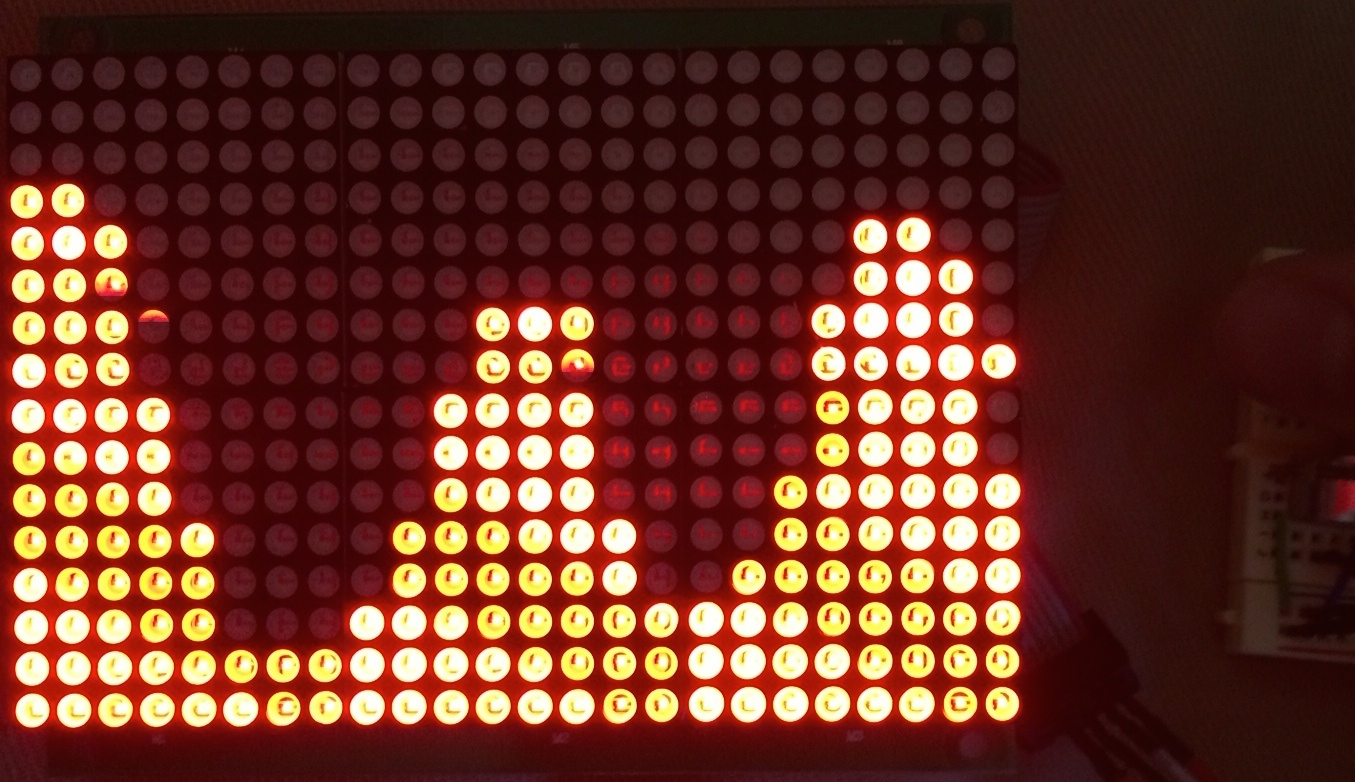Build this project at our next weekend-long Arduino workshop!# LED Matrix Graph

LED Matrix Graph is a simple program to track two physical values over time. Start with two potentiometers; variations replace the potentiometers with Light-dependent Resistors (LDRs), then with capacitive sensors. The two values are graphically represented on a 16x24 Red LED Matrix, like this one from Adafruit.

Requires minimal soldering skill; moderately complex programming.

## Hardware

You’ll need the following components:

Preparing the LED Matrix board may also require a soldering iron to bridge jumper J5 on the back of the board. See the Adafruit tutorial for details.

## Wiring

1. Build this circuit:2. Plug jumper wires into the ribbon cable and your Arduino as shown in this Adafruit tutorial, but plug the 5V (red) and GND (black) wires into your breadboard instead of directly into the Arduino. Try to match colors if you can; it will make for less (and eaiser) debugging.

## Software

1. Download and install the HT1632 library for the LED matrix board.

2. Copy this code into a new Arduino sketch:

``````// LED Matrix Graph
// by J.D. Zamfirescu
// Last update: June 2014
// graph.ino

#include "HT1632.h" // Get this library here: http://github.com/workshopweekend/HT1632

#define DATA 2
#define WR   3
#define CS   4

HT1632LEDMatrix matrix = HT1632LEDMatrix(DATA, WR, CS);

const int numSamples = 24;

int a_values[numSamples], b_values[numSamples];
int ptr = 0;

void setup() {
matrix.begin(HT1632_COMMON_16NMOS);
matrix.clearScreen();
Serial.begin(9600);
}

void loop() {
matrix.setScreen(0);
for (int i = 0; i < numSamples; i++) {
matrix.drawLine(i, b_values[(ptr+i)%numSamples], i, a_values[(ptr+i)%numSamples], 1);
}
matrix.writeScreen();
a_values[ptr] = getAValue();
b_values[ptr] = getBValue();
ptr = (ptr + 1) % numSamples;
delay(50);
}

int getAValue() {
}

int getBValue() {
}
``````

## Variations

1. Replace the potentiometers with a voltage divider consisting of a light-dependent resistor and a 10 kΩ resistor, as in the following circuit:Note that the LDR circuits now never reach either extreme of their range. Read about the `map` function and see if you can resolve this issue.

2. Replace the LDRs with capacitive sensing inputs, using a 1 MΩ resistor as in the following circuit:Then, replace the `getAValue` and `getBValue` functions with the following code:

``````#include <CapacitiveSensor.h>

CapacitiveSensor sensorA = CapacitiveSensor(10,9);
CapacitiveSensor sensorB = CapacitiveSensor(10,11);

int getAValue() {
return sensorA.capacitiveSensor(25)/64;
}

int getBValue() {
return sensorB.capacitiveSensor(25)/64;
}
``````